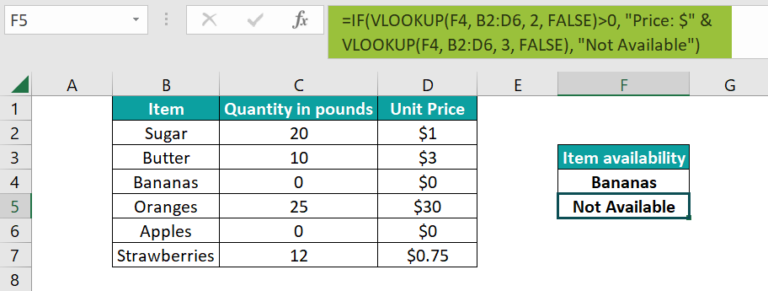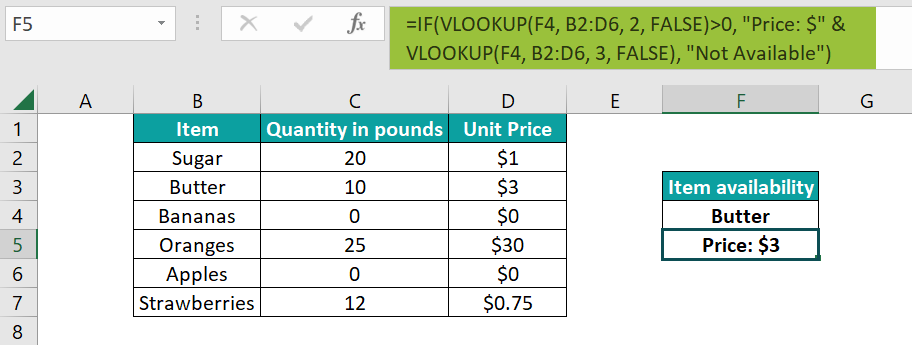# VLOOKUP with IF StatementArticle byExcelMojo Team## What is VLOOKUP with IF Statement in Excel?

VLOOKUP is one of the most powerful functions in Excel. It is used to look up data in a table that is organized vertically. Here, a specific value is looked up in one table column and returns the corresponding value from another. When we combine VLOOKUP with IF Statement, it helps check for specified conditions making it a powerful combination.

For instance, below is a table containing the names of 3 students. We must look up their marks and announce the result accordingly. Enter the formula =IF(VLOOKUP(“Danny”, A1:B4,2, FALSE) > 50, “Pass”, “Fail”) in cell B5. Here we are looking up the name of Danny in Column A and then finding his corresponding marks. If it exceeds 50, he is declared “Pass”; else, “Fail.”

###### Key Takeaways
• The combination of VLOOKUP with IF statement helps find values that would otherwise be impossible to obtain. If the lookup value is not found, we can use the IFNA statement to handle the N/A error.
• The most common use of the VLOOKUP statement with IF is to compare the value VLOOKUP returns with a sample value and return True/False based on the outcome.
• The INDEX MATCH combo can be used to search from right to left instead of VLOOKUP. You can also use the XLOOKUP function.

### VLOOKUP with IF() Excel Function

Before we check the syntax of the VLOOKUP with IF, let us look at the formula for VLOOKUP.

Arguments

• lookup value (mandatory) – The cell value or a text that we are looking for
• table_array (mandatory) – The range of cells where we look for the value.
• col_index_number – Column index number from which you want the result
• range_lookup – True or false to get the exact match or approximate data.

The combined syntax of the formula of VLOOKUP and IF is as follows:

Here, we look up a particular value and check if it satisfies a given condition. Then, it returns TRUE, or else FALSE.

### How to Use VLOOKUP with IF Statement Function in Excel?

The VLOOKUP with IF statement can be entered in an Excel cell as a formula based on our requirement. First, let us look at how to do this step-by-step.

In this example, we have the inventory details of a supermarket. Here, the table contains a list of products and their quantity with the price. Next, we must check if an item is available and, if so, what its price is.

The steps to use VLOOKUP with IF statement is as follows –

1. First, we must check whether an item is available. If available, we must display its unit price. Now, enter the item to find in cell F4. For instance, let us type the item “Bananas.”

Enter the following formula in cell F5.

=IF(VLOOKUP(F4, B2:D6, 2, FALSE)>0, “Price: \$” & VLOOKUP(F4, B2:D6, 3, FALSE), “Not Available”)

Here,

We use multiple VLOOKUP with IF Statement. The first VLOOKUP in the IF condition, checks if the value entered in F4 is present in the range and, if so, what its price is.
‘2’ specifies Column C, the second column in the range; it contains the return value.
If the quantity is larger than zero, we display the price. For this, we use the VLOOKUP function again to get the corresponding cost from Column D, which is the third column.
If the quantity is zero, we get the message, “Not available.”2. As observed, when we used the formula for the value “Bananas,” we got the message “Not Available” since the number of bananas in the inventory was zero. So now, let us change the entry in D4 to “Butter” and check the result.We can see that since butter is present in the inventory list, its price is displayed.

Thus, the VLOOKUP with IF statement can be written in any form to suit our requirements.

### Examples

The full power of the VLOOKUP function can be extracted by combining it with the IF function in Excel. Here are some examples of how this combination can extract information from any table.

#### Example #1

• VLOOKUP with IF statement based on the Search Result

In this example, we have two tables. Table 1 contains the names and scores of some students in Grade 4, while Table 2 contains the same information about some students in Grade 5. Now, we must enter the name and grade of a student and display their score in cell B11.

• Step 1: Enter the student’s name and grade in cells B9 and B10, respectively. Enter the following formula to find the score in cell B11:

• We check if the Grade entered is Grade 4 using the IF statement.
• If so, we use the first VLOOKUP to scout the first table for the name specified in B9 and return the score.
• If it isn’t Grade 4, we use the VLOOKUP to scout the second table and find the student’s name and their corresponding score. ‘

For now, we specify the entries in B9 and B10 as Mindy and Grade 4, respectively. Press Enter after typing the formula given above.

• Step 2: The score of Mindy has been picked from the first table and is displayed in B11. Apply the same formula by changing the B9 and B10 entries to Julia and Grade 5.

We observe that there are 2 Julias in grades 4 and 5. However, since the IF condition returns false, it executes the second VLOOKUP and searches the second table for Grade 5.

Thus, VLOOKUP with IF statement can be used in many complex searches.

#### Example #2

• Dynamic Selection of Column Index Argument

Let us look at an example where we select the column index dynamically. Below is a table containing some of the household expenses of an individual in March and April. First, we must choose an item. Then, based on the month chosen, the expense will be displayed. We will use Data Validation in excel to select the month.

• Step 1: We have the below table.
• Cell C11 has been modified to contain a list of either March or April as choices.
• For this, proceed to the Data tab and choose Data Validation. In the Settings tab, select List in the Allow box.
• Click in the Source box, then select your list range. Here, we must choose between March and April, so we selected cells C3 and C4. Press OK.

You now have a list in cell C11 with the options March/April.

• Step 2: Enter the name “Fuel” in cell C10 and choose April from the list in C11. Now, type the below formula in cell C12. Press Enter.

=VLOOKUP(C10, B2:D8, IF(C11 = “March”, 2, 3), FALSE)

You get the Fuel expenses of \$52 for April.

Explanation:

• In this formula, we look up the value in cell C10, Fuel in this case, in the data range of the table.
• Based on our choice in cell C11, the column index is assigned using the IF statement in argument 3. If the month is March, the column index is two, else three.
• Thus, using VLOOKUP with IF statement displays the appropriate expenses.

#### Example #3

• Using IFNA with VLOOKUP with IF statement

We have the names of some employees of a firm and their bonuses. Therefore, when we enter a name in cell E4, their performance will be rated based on their bonus.

Step 1: We use the VLOOKUP with IF statement in this scenario. Enter the following formula in cell F5.

=IF(VLOOKUP(E4, B3:C7, 2, FALSE)>2000, “Amazing!!”, “Good”)

• This formula helps search for the name specified in E4 and then find the corresponding bonus using the column index.
• If it is greater than \$2,000, we get “Amazing!!” as the output, else “Good.”

Now, if we enter a name like Peter in E4, we get an #N/A error as the name is not in the specified range.

• Step 2: To overcome this issue, we can use the IFNA function, which executes specific actions based on the condition provided. Alter the formula in F4 as follows, and press Enter for the same entry Peter.

• Here, we add the IFNA function around our existing formula, which in case of no error, just prints the output, else prints “Not found.”

Thus, the problem of the N/A error can be solved using IFNA with VLOOKUP and IF statement.

### Important Things to Note

• When you use VLOOKUP, you get the N/A error whenever the value is not found. To trap any error, not just the IFNA function, you can use VLOOKUP with the IFERROR function.
• Remember, VLOOKUP cannot search from right to left, only left to right. For searching in either direction, the INDEX MATCH combo or the XLOOKUP function can be used.
• Before the compact IFNA, the IF ISNA was used to check for errors.
• In VLOOKUP, the fourth argument TRUE means an approximate match while FALSE means an exact match.

1. Why is VLOOKUP with IF statement not working?

The VLOOKUP, when used with IF, would give a N/A error if the item specified in the search criteria of VLOOKUP does not exist. Further, when using the IF or VLOOKUP function, you should ensure that there are three arguments(at least three in the case of VLOOKUP) to avoid #VALUE errors.

2. What are the three required arguments in VLOOKUP with IF statement?

The first argument in the IF statement is the VLOOKUP formula, which returns a search value. Then, you must specify a condition for this return value. If the statement is TRUE, you give a particular output; if FALSE, you get another. Thus, VLOOKUP with IF Statement yes or no, true or false, is used.

3. What are the two main causes of errors for VLOOKUP with IF statement?

If either of the functions, VLOOKUP or IF, has fewer arguments, you get an #VALUE error.
If the lookup value is not found in the VLOOKUP function, you get a N/A error.

4. What are some limitations of VLOOKUP with IF statement?

One of the limitations is that you will not get an output in case of an error.
VLOOKUP does not allow multiple criteria and a right-to-left search.